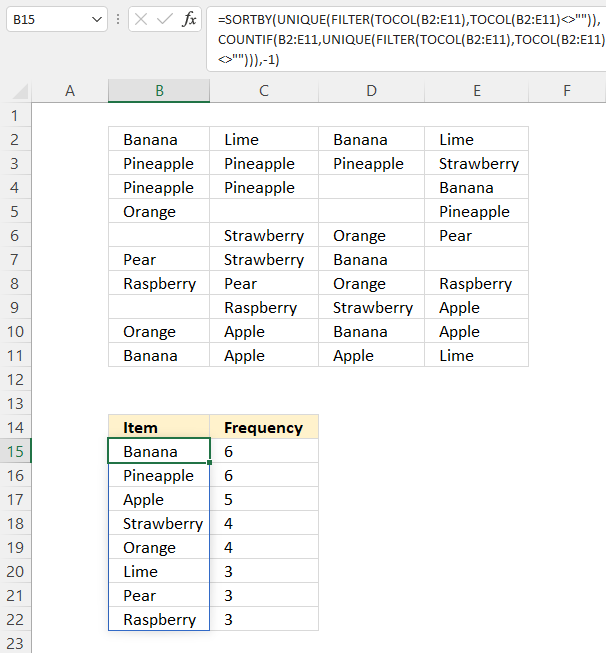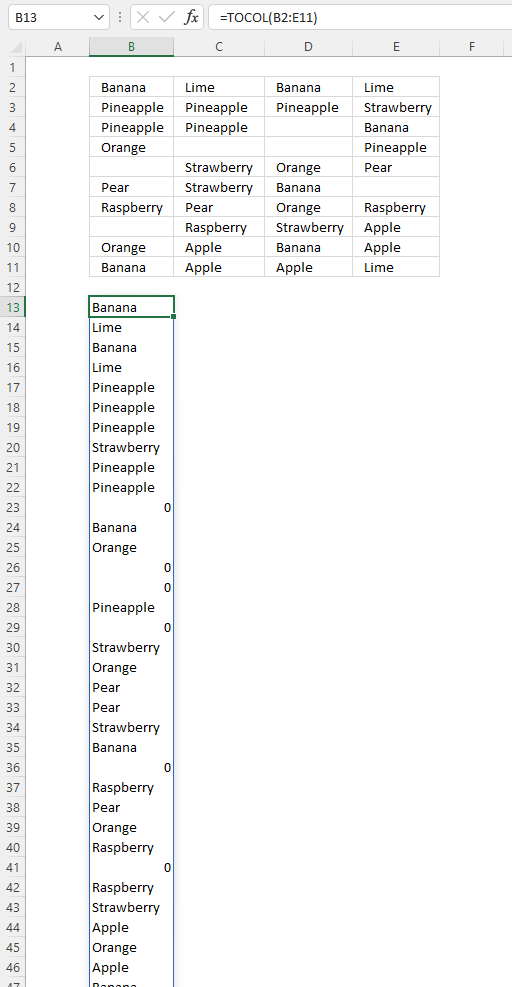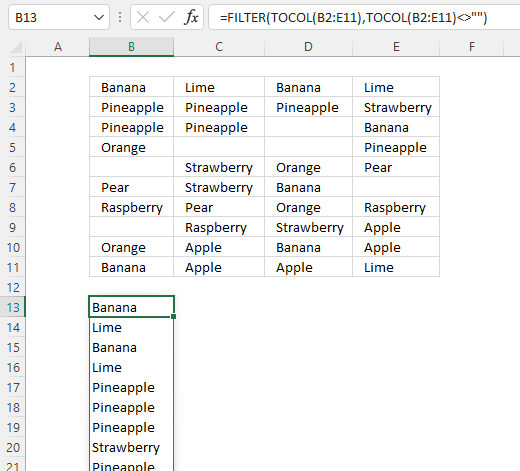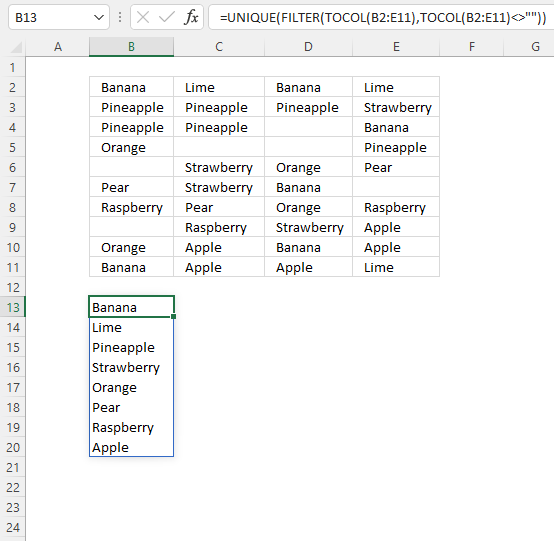Author: Oscar Cronquist Article last updated on May 11, 2022Cell range B2:E11 contains values, the formula in cell B15 extracts unique distinct values in B2:E11, ignores blanks, and returns a list sorted based on frequency.

Dynamic array formula in cell B15:

=LET(x,B2:E11,y,TOCOL(x),z,UNIQUE(FILTER(y,y<>"")),SORTBY(z, COUNTIF(x,z),-1))

### Explaining formula

#### Step 1 - Rearrange values to a single column

The TOCOL function rearranges values in a 2D cell range to a single column.

TOCOL(array, [ignore], [scan_by_col])

TOCOL(B2:E11)

returns#### Step 2 - Filter out blanks

The FILTER function extracts values/rows based on a condition or criteria.

FILTER(arrayinclude, [if_empty])

FILTER(TOCOL(B2:E11), TOCOL(B2:E11)<>"")

returnsThe empty cells are now gone in cell B13 and cells below.

#### Step 3 - Extract unique values

The UNIQUE function extracts both unique and unique distinct values and also compare columns to columns or rows to rows.

UNIQUE(array,[by_col],[exactly_once])

UNIQUE(FILTER(TOCOL(B2:E11), TOCOL(B2:E11)<>""))

returns

{"Banana"; "Lime"; "Pineapple"; "Strawberry"; "Orange"; "Pear"; "Raspberry"; "Apple"}.#### Step 4 - Count values

The COUNTIF function calculates the number of cells that meet a given condition.

COUNTIF(rangecriteria)

COUNTIF(B2:E11, UNIQUE(FILTER(TOCOL(B2:E11), TOCOL(B2:E11)<>"")))

becomes

COUNTIF(B2:E11, {"Banana"; "Lime"; "Pineapple"; "Strawberry"; "Orange"; "Pear"; "Raspberry"; "Apple"})

and returns

{6; 3; 6; 4; 4; 3; 3; 5}.

#### Step 5 - Sort values based on the frequency

The SORTBY function sorts values from a cell range or array based on a corresponding cell range or array.

SORTBY(array, by_array1, [sort_order1], [by_array2, sort_order2],…)

SORTBY(UNIQUE(FILTER(TOCOL(B2:E11), TOCOL(B2:E11)<>"")), COUNTIF(B2:E11, UNIQUE(FILTER(TOCOL(B2:E11), TOCOL(B2:E11)<>""))), -1)

becomes

SORTBY({"Banana"; "Lime"; "Pineapple"; "Strawberry"; "Orange"; "Pear"; "Raspberry"; "Apple"}, {6; 3; 6; 4; 4; 3; 3; 5}, -1)

and returns

{"Banana"; "Pineapple"; "Apple"; "Strawberry"; "Orange"; "Lime"; "Pear"; "Raspberry"}.

#### Step 6 - Shorten the formula

The LET function lets you name intermediate calculation results which can shorten formulas considerably and improve performance.

LET(name1name_value1calculation_or_name2, [name_value2calculation_or_name3...])

SORTBY(UNIQUE(FILTER(TOCOL(B2:E11), TOCOL(B2:E11)<>"")), COUNTIF(B2:E11, UNIQUE(FILTER(TOCOL(B2:E11), TOCOL(B2:E11)<>""))), -1)

x - B2:E11
y - TOCOL(x)
z - UNIQUE(FILTER(y,y<>""))

LET(x,B2:E11,y,TOCOL(x),z,UNIQUE(FILTER(y,y<>"")),SORTBY(z, COUNTIF(x,z),-1))